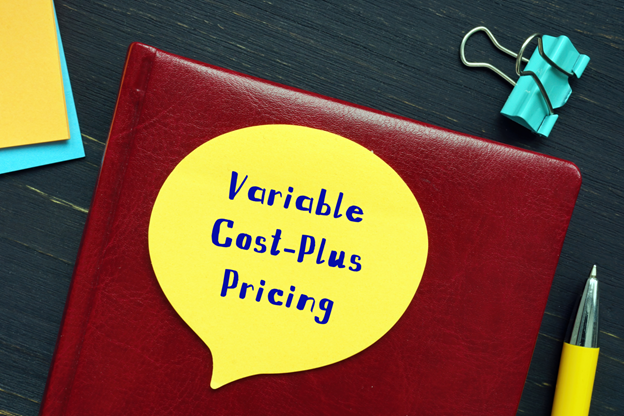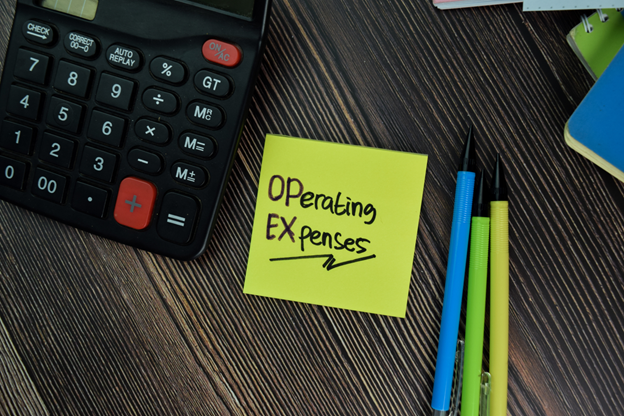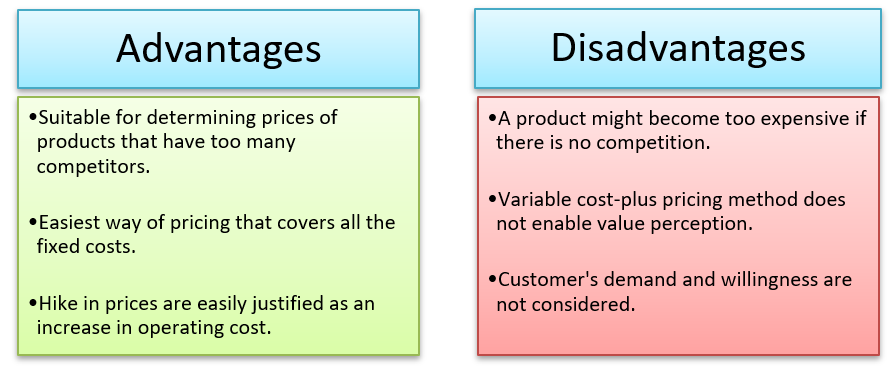# Variable Cost-Plus Pricing

• Updated on

## What is variable cost-plus pricing?

Variable cost-plus pricing can be defined as a type of pricing method where the selling price is determined by adding the difference between the lowest current price offered amongst the broker-dealer and the price charged to the customers for the investment as markup to total variable cost. The sole purpose behind variable cost-plus pricing is to generate some revenue even after covering all the operating expenses and overheads.

Summary
• Variable cost-plus pricing refers to the type of pricing method where the selling price is determined by adding the markup to the total cost of a product or service.
• The objective of variable cost-plus pricing is to generate revenue to cover all the operating expenses, variable costs, and overheads.
• The selling price of an item can be easily determined using the variable cost-plus pricing method by multiplying the total unit cost of an item with a markup percentage.

## How to analyse the variable cost-plus pricing?

Variable cost refers to the operating expenses subject to change with the increase or decrease in production. For example, the cost of raw materials, labour charge, packing cost, etc., come under variable cost. Therefore, the companies who have a high chance of incurring variable costs must employ a variable cost-plus pricing method while setting the prices of their products.

These steps must follow to employ the variable cost-plus pricing:

Step 1- Obtain the total cost of production for a particular service or product. Then, find out the sum of fixed cost and variable cost to obtain the total cost.

Step 2- Obtain the unit cost of the product or service by dividing the total cost by the number of manufactured products.

Step 3- Set the selling price by multiplying the unit cost that has been obtained earlier with the markup percentage.Image source: © Rummess | Megapixl.com

## How does the variable cost-plus pricing work?

Many companies incur a high variable cost, including the labor charge, cost of raw materials, etc., that vary with the production quantity. Therefore, such companies must employ the variable cost-plus pricing method to determine the prices of their services and products. To implement the variable cost-plus pricing method, the company must calculate its variable cost precisely beforehand to determine the expected profit from the number of units produced.

For example, let us assume that the operating expense of a particular product is AU\$5. Now the company calculates that the fixed cost for manufacturing the product is AU\$3. Therefore, to compensate for the fixed cost, the profit set by the company is AU\$2. Thus the selling price of the product is set to be AU\$ (5+3+2) = AU\$ 10.Image source: © Olandah23 | Megapixl.com

## What are the practical applications of variable cost-plus pricing?

The variable cost-plus pricing method is ideal for companies with a high portion of the total cost as a variable cost. By employing this method, these companies can ensure that it will compensate both fixed and variable costs incurred while manufacturing the products. However, suppose the variable cost ratio to the fixed cost is low. In that case, it indicates that the company experiences fixed cost more than variable cost, and applying the variable cost-plus pricing method in such case could result in inaccuracy in prices and unsustainability to earning revenue in the long run.

The application of the variable cost-plus pricing method is also widely found in companies with excess capacity. In such companies, fixed costs would not go up with the increase in the production quantity (for example, no extra money would be spent upon warehouse rent). Therefore, the variable cost in such cases would directly be proportional to the production quantity. Hence, the markup percentage could be added to the unit variable cost to set the selling price to offer a decent profit to the company.The following are the advantages of variable cost-plus pricing:

• Variable cost-plus pricing is very beneficial in the competitive market, such as contract bidding.
• Variable cost-plus pricing is one of the simplest methods of setting the selling price of an item that quickly covers the fixed and variable costs.
• It is easier to lock revenue with contracts by applying the method of variable cost-plus pricing. This is because every supplier mostly prefers contracts with dealers who guarantee sales with a set profit level and ensures that all production costs are compensated. It also eliminates all the possible chances of loss due to poor pricing strategies.
• It also becomes easier for suppliers to justify price increase with the help of variable cost-plus pricing as it can be explained as the increase in the cost of operating expenses.

The following are the disadvantages involved with variable cost-plus pricing:

• There might be situations in which the variable cost-plus pricing would not consider the competition. In such a situation, a particular product may end up being highly priced despite other brands in the market selling a similar product at a lower price and vice versa.
• The perception of the value and price of a particular product comparing to its competition is not possible with the variable cost-plus pricing method. While setting the selling price of a product, companies should also consider customer's desire to purchase the item.

## What could be an ideal example of variable cost-plus pricing?

Let us assume that the manufacturer quotes the price of speakers using the variable cost-plus pricing method. The variable cost involved in manufacturing a single speaker is AU\$20, and the markup percentage is 40. Therefore, the selling price can be calculated using the variable cost-plus pricing method:

Selling price = 20*1.4 = AU\$28

On the other hand, the fixed cost involved with the manufacture of a single speaker is AU\$5. So the total cost of producing a single unit of a speaker is AU\$(20+5)= AU\$25. Since the selling price is quoted as AU\$28, the company earns a profit of AU\$3 for the sale of each speaker.

##### Top ASX Listed Companies

Definition:
We use cookies to ensure that we give you the best experience on our website. If you continue to use this site we will assume that you are happy with it. OK